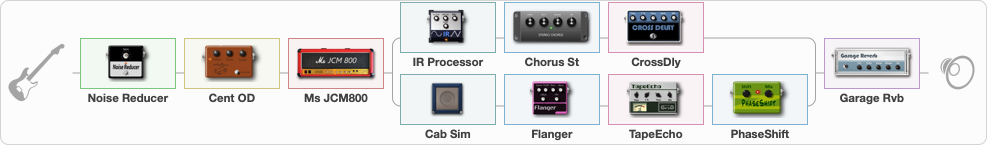# VH Old School

Discussion in 'ToneLib-GFX presets' started by davidtaylors, Mar 19, 2020.

1. ### davidtaylorsWell-Known Member

VH Old School

Preset name: VH Old School

Going old school on this one. Drop dead Legs to 5150

Effects chain:Effect: "Noise Reducer" (Dynamics / Filter), active - "yes"
{
"Sens" = 81
"Mode" = Soft
}

Effect: "Cent OD" (Overdrive / Distortion), active - "yes"
{
"Drive" = 52
"Tone" = 70
"Level" = 74
}

Effect: "Ms JCM800" (Amp simulators), active - "yes"
{
"Gain" = 100
"Bass" = 82
"Middle" = 23
"Treble" = 39
"Presence" = 65
"Master" = 78
"Level (dB)" = 2
}

Effect: "Splitter" (Dynamics / Filter), active - "yes"
{
"A-Bypass" = Off
"A-Pan" = -42
"A-Level" = 55
"B-Bypass" = Off
"B-Pan" = 50
"B-Level" = 55

'A' branch:
{

Effect: "IR Processor" (Cabinets), active - "yes"
{
"IR" = Cab IR Sample1
"Low Cut (Hz)" = 0
"Hi Cut (kHz)" = 20.0
"Mix" = 100
"Level (dB)" = 10
}

Effect: "Chorus St" (Modulation / Sfx), active - "yes"
{
"Speed" = 1.9
"Depth" = 31
"Center" = 4.4
"Mix" = 40
}

Effect: "CrossDly" (Delay), active - "yes"
{
"Time" = 756
"Feedback" = 27
"Tone" = 57
"Sens" = 29
"Mix" = 34
}
}
'B' branch:
{

Effect: "Cab Sim" (Cabinets), active - "yes"
{
"Model" = 4x12" 1960 T75
"Level (dB)" = 7
}

Effect: "Flanger" (Modulation / Sfx), active - "yes"
{
"Speed" = 0.8
"Depth" = 71
"Reso" = 35
"Center" = 7.7
"Offset" = 0.0
"Mix" = 57
"Reset" = On
}

Effect: "TapeEcho" (Delay), active - "yes"
{
"Time" = 257
"Feedback" = 38
"Tone" = 65
"LoDamp" = 19
"Mix" = 65
}

Effect: "PhaseShift" (Modulation / Sfx), active - "yes"
{
"Shift" = 50
"Mix" = 64
}
}
}

Effect: "Garage Rvb" (Reverb), active - "yes"
{
"Time" = 3.3
"PreDelay" = 29
"LoDamp" = 0
"HiDamp" = 30
"Mix" = 61
}

Note: You will need to download and install the ToneLib-GFX software to use the preset.

#### Attached Files:

• ###### VH_Old_School.tlgfx
File size:
5.2 KB
Views:
9,553
2. Great! Nice attention to detail! Which IR did you decide on for the Left channel? Cheers!

3. I agree. This preset is sweet. Thank you for sharing.